# A Comparison of Linear Gradients in Different Colourspaces

by Billy Biggs <vektor@dumbterm.net>

The following is a comparison of gradients generated in different colourspaces. The colours for the endpoints are defined in linear sRGB space. They were chosen such that none of the resulting colours were out of gamut in each of the colour spaces we tested with. They are listed in the table below. All of the gradients are generated assuming sRGB viewing conditions.

SourceDestination
Red Green Blue Red Green Blue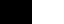(0.0, 0.0, 0.0) (1.0, 1.0, 1.0)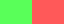(0.1, 1.0, 0.1) (1.0, 0.1, 0.1)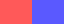(1.0, 0.1, 0.1) (0.1, 0.1, 1.0)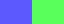(0.1, 0.1, 1.0) (0.1, 1.0, 0.1)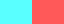(0.1, 1.0, 1.0) (1.0, 0.1, 0.1)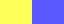(1.0, 1.0, 0.1) (0.1, 0.1, 1.0)

Here are the linear in intensity gradients.## RGB gradients not gamma corrected

Here are the gradients not gamma corrected. These are the gradients you get when you interpolate the pixel values.Ok I lied, I am just doing a cube and cube root, but by the CIELAB equations this is equivalent.